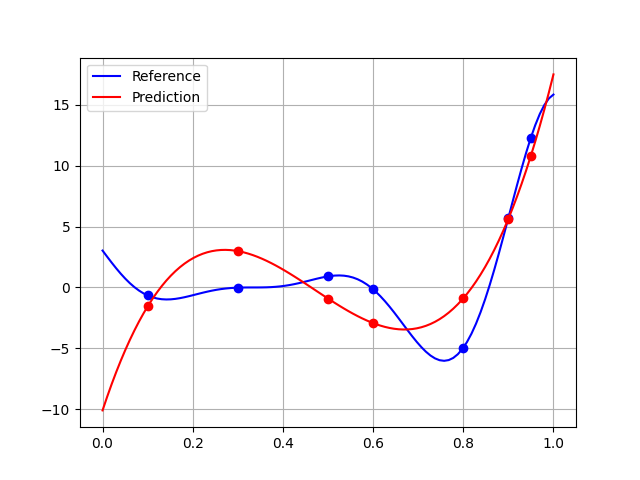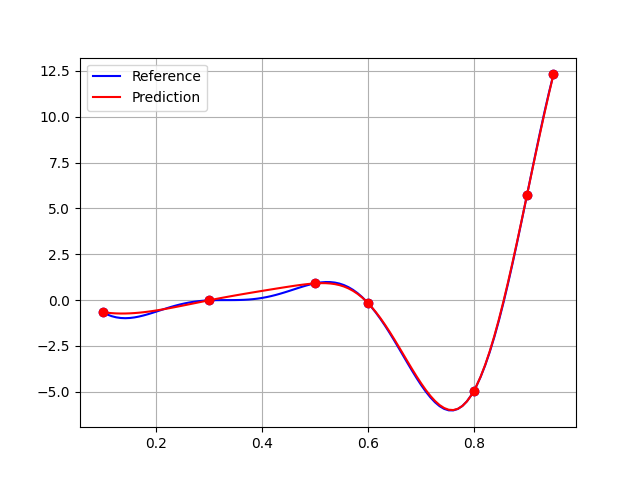# MSE example - test-train split¶

In this example we consider a polynomial linear regression, splitting the data into two sets. We measure the quality of the regression by comparing the predictions with the output on the test set.

from __future__ import division, unicode_literals

import matplotlib.pyplot as plt
from numpy import arange, argmin, hstack, linspace, sort
from numpy.random import choice, normal, seed

from gemseo.api import configure_logger, create_dataset
from gemseo.core.dataset import Dataset
from gemseo.mlearning.api import create_regression_model
from gemseo.mlearning.qual_measure.mse_measure import MSEMeasure

configure_logger()


Out:

<RootLogger root (INFO)>


## Define parameters¶

seed(12345)
n_samples = 10
noise = 0.3 ** 2
max_pow = 5
amount_train = 0.8


## Construct data¶

We construct a parabola with added noise, on the interval [0, 1].

def f(x):
return -4 * (x - 0.5) ** 2 + 3

x = linspace(0, 1, n_samples)
y = f(x) + normal(0, noise, n_samples)


## Indices for test-train split¶

samples = arange(n_samples)
n_train = int(amount_train * n_samples)
n_test = n_samples - n_train
train = sort(choice(samples, n_train, False))
test = sort([sample for sample in samples if sample not in train])
print("Train:", train)
print("Test:", test)


Out:

Train: [1 3 4 5 6 7 8 9]
Test: [0 2]


## Build datasets¶

data = hstack([x[:, None], y[:, None]])
variables = ["x", "y"]
groups = {"x": Dataset.INPUT_GROUP, "y": Dataset.OUTPUT_GROUP}
dataset = create_dataset("synthetic_data", data[train], variables, groups=groups)
dataset_test = create_dataset("synthetic_data", data[test], variables, groups=groups)


## Build regression model¶

model = create_regression_model("PolynomialRegression", dataset, degree=max_pow)
print(model)


Out:

PolynomialRegression(degree=5, fit_intercept=True, l2_penalty_ratio=1.0, penalty_level=0.0)
based on the scikit-learn library


## Predictions errors¶

measure = MSEMeasure(model)

mse_train = measure.evaluate("learn")
mse_test = measure.evaluate("test", test_data=dataset_test)

print("Training error:", mse_train)
print("Test error:", mse_test)


Out:

/home/docs/checkouts/readthedocs.org/user_builds/gemseo/conda/stable/lib/python3.8/site-packages/sklearn/linear_model/_base.py:148: FutureWarning: 'normalize' was deprecated in version 1.0 and will be removed in 1.2. Please leave the normalize parameter to its default value to silence this warning. The default behavior of this estimator is to not do any normalization. If normalization is needed please use sklearn.preprocessing.StandardScaler instead.
warnings.warn(
/home/docs/checkouts/readthedocs.org/user_builds/gemseo/conda/stable/lib/python3.8/site-packages/sklearn/linear_model/_base.py:148: FutureWarning: 'normalize' was deprecated in version 1.0 and will be removed in 1.2. Please leave the normalize parameter to its default value to silence this warning. The default behavior of this estimator is to not do any normalization. If normalization is needed please use sklearn.preprocessing.StandardScaler instead.
warnings.warn(
Training error: [0.0003947]
Test error: [2.29565983]


## Compute predictions¶

measure = MSEMeasure(model)
model.learn()

n_refined = 1000
x_refined = linspace(0, 1, n_refined)
y_refined = model.predict({"x": x_refined[:, None]})["y"].flatten()


Out:

/home/docs/checkouts/readthedocs.org/user_builds/gemseo/conda/stable/lib/python3.8/site-packages/sklearn/linear_model/_base.py:148: FutureWarning: 'normalize' was deprecated in version 1.0 and will be removed in 1.2. Please leave the normalize parameter to its default value to silence this warning. The default behavior of this estimator is to not do any normalization. If normalization is needed please use sklearn.preprocessing.StandardScaler instead.
warnings.warn(


## Plot data points¶

plt.plot(x_refined, f(x_refined), label="Exact function")
plt.scatter(x, y, label="Data points")
plt.legend()
plt.show()## Plot predictions¶

plt.plot(x_refined, y_refined, label="Prediction (x^{})".format(max_pow))
plt.scatter(x[train], y[train], label="Train")
plt.scatter(x[test], y[test], color="r", label="Test")
plt.legend()
plt.show()## Compare different parameters¶

powers = [1, 2, 3, 4, 5, 7]
test_errors = []
for power in powers:
model = create_regression_model("PolynomialRegression", dataset, degree=power)
measure = MSEMeasure(model)

test_mse = measure.evaluate("test", test_data=dataset_test)
test_errors += [test_mse]

y_refined = model.predict({"x": x_refined[:, None]})["y"].flatten()

plt.plot(x_refined, y_refined, label="x^{}".format(power))

plt.scatter(x[train], y[train], label="Train")
plt.scatter(x[test], y[test], color="r", label="Test")
plt.legend()
plt.show()Out:

/home/docs/checkouts/readthedocs.org/user_builds/gemseo/conda/stable/lib/python3.8/site-packages/sklearn/linear_model/_base.py:148: FutureWarning: 'normalize' was deprecated in version 1.0 and will be removed in 1.2. Please leave the normalize parameter to its default value to silence this warning. The default behavior of this estimator is to not do any normalization. If normalization is needed please use sklearn.preprocessing.StandardScaler instead.
warnings.warn(
/home/docs/checkouts/readthedocs.org/user_builds/gemseo/conda/stable/lib/python3.8/site-packages/sklearn/linear_model/_base.py:148: FutureWarning: 'normalize' was deprecated in version 1.0 and will be removed in 1.2. Please leave the normalize parameter to its default value to silence this warning. The default behavior of this estimator is to not do any normalization. If normalization is needed please use sklearn.preprocessing.StandardScaler instead.
warnings.warn(
/home/docs/checkouts/readthedocs.org/user_builds/gemseo/conda/stable/lib/python3.8/site-packages/sklearn/linear_model/_base.py:148: FutureWarning: 'normalize' was deprecated in version 1.0 and will be removed in 1.2. Please leave the normalize parameter to its default value to silence this warning. The default behavior of this estimator is to not do any normalization. If normalization is needed please use sklearn.preprocessing.StandardScaler instead.
warnings.warn(
/home/docs/checkouts/readthedocs.org/user_builds/gemseo/conda/stable/lib/python3.8/site-packages/sklearn/linear_model/_base.py:148: FutureWarning: 'normalize' was deprecated in version 1.0 and will be removed in 1.2. Please leave the normalize parameter to its default value to silence this warning. The default behavior of this estimator is to not do any normalization. If normalization is needed please use sklearn.preprocessing.StandardScaler instead.
warnings.warn(
/home/docs/checkouts/readthedocs.org/user_builds/gemseo/conda/stable/lib/python3.8/site-packages/sklearn/linear_model/_base.py:148: FutureWarning: 'normalize' was deprecated in version 1.0 and will be removed in 1.2. Please leave the normalize parameter to its default value to silence this warning. The default behavior of this estimator is to not do any normalization. If normalization is needed please use sklearn.preprocessing.StandardScaler instead.
warnings.warn(
/home/docs/checkouts/readthedocs.org/user_builds/gemseo/conda/stable/lib/python3.8/site-packages/sklearn/linear_model/_base.py:148: FutureWarning: 'normalize' was deprecated in version 1.0 and will be removed in 1.2. Please leave the normalize parameter to its default value to silence this warning. The default behavior of this estimator is to not do any normalization. If normalization is needed please use sklearn.preprocessing.StandardScaler instead.
warnings.warn(


Grid search

print(test_errors)
print("Power for minimal test error:", argmin(test_errors))


Out:

[array([0.54513687]), array([0.00518409]), array([0.00584647]), array([0.06387849]), array([2.29565983]), array([1.16961302])]
Power for minimal test error: 1


Total running time of the script: ( 0 minutes 0.434 seconds)

Gallery generated by Sphinx-Gallery